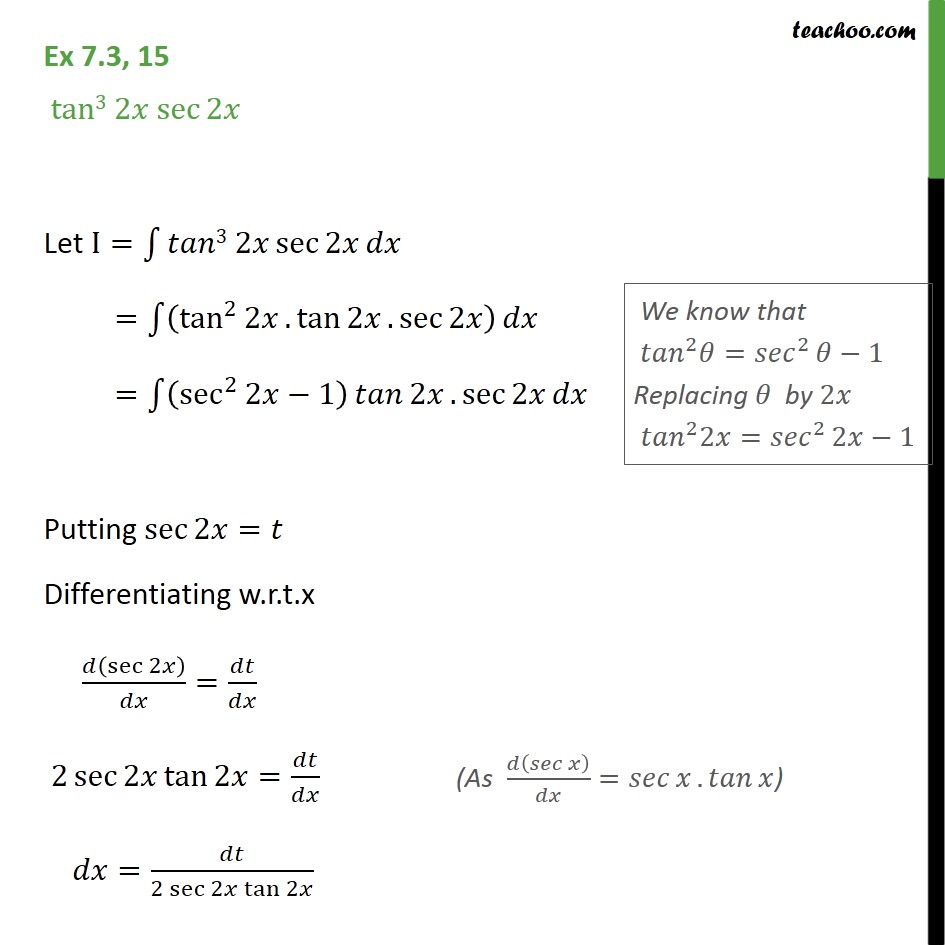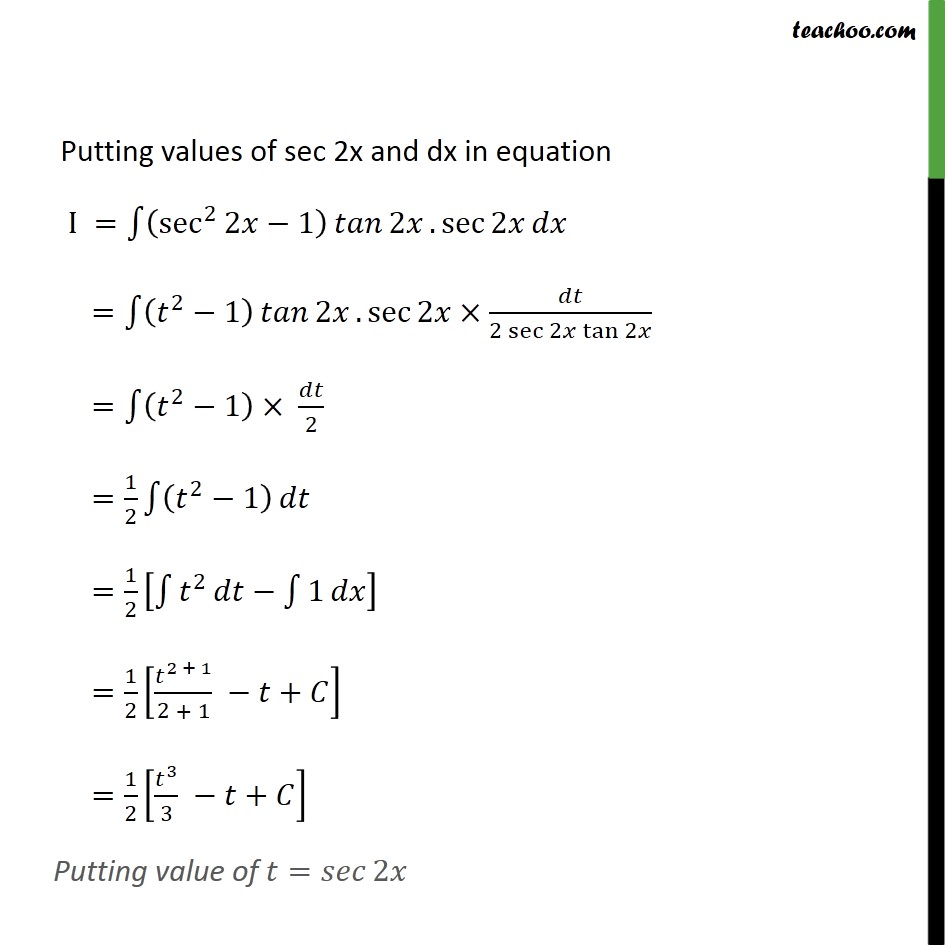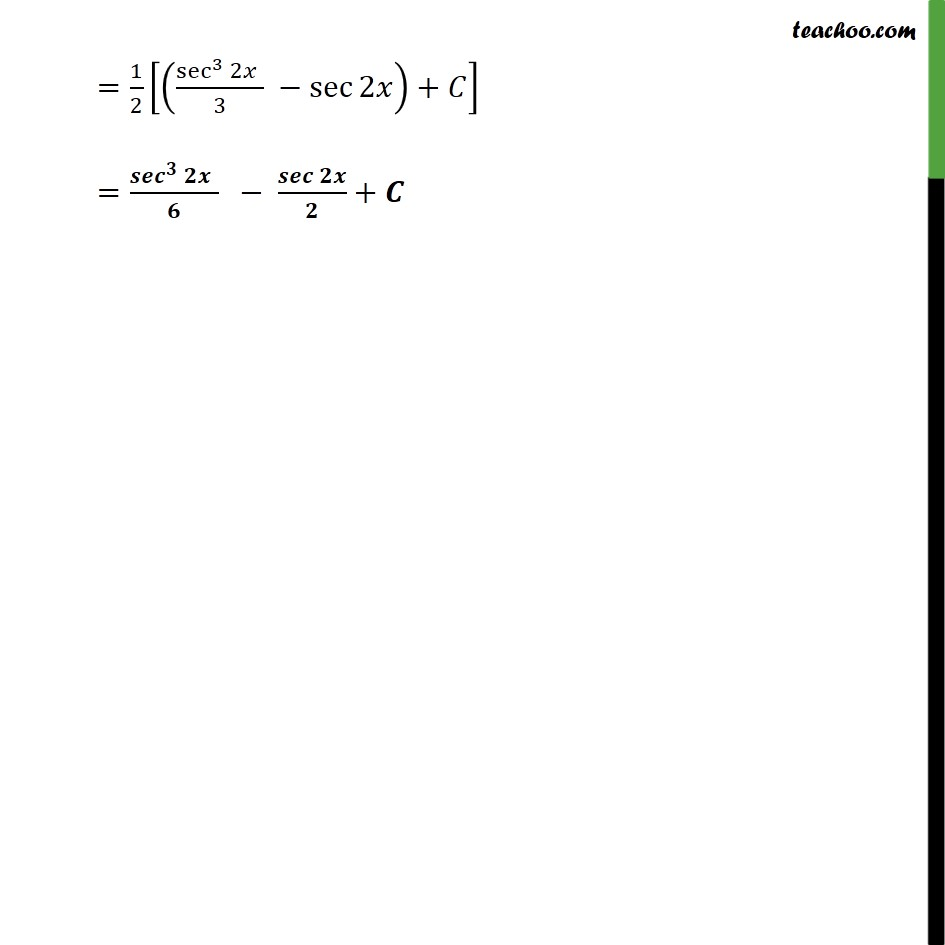Integration using trigo identities - sin^2,cos^2 etc formulae

Chapter 7 Class 12 Integrals
Concept wiseIntroducing your new favourite teacher - Teachoo Black, at only ₹83 per month

### Transcript

Ex 7.3, 15 tan3 2 sec 2 Let I= 3 2 sec 2 = tan 2 2 . tan 2 . sec 2 = sec 2 2 1 2 . sec 2 Putting sec 2 = Differentiating w.r.t.x sec 2 = 2 sec 2 tan 2 = = 2 sec 2 tan 2 Putting values of sec 2x and dx in equation I = sec 2 2 1 2 . sec 2 = 2 1 2 . sec 2 2 sec 2 tan 2 = 2 1 2 = 1 2 2 1 = 1 2 2 1 = 1 2 2 + 1 2 + 1 + = 1 2 3 3 + Putting value of = 2 = 1 2 sec 3 2 3 sec 2 + = +